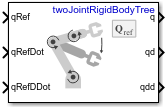Joint Space Motion Model

Model rigid body tree motion given joint-space inputs

• Library:
• Robotics System Toolbox / Manipulator Algorithms

•Description

The Joint Space Motion Model block models the closed-loop joint-space motion of a manipulator robot, specified as a rigidBodyTree object. The motion model behavior is defined by the Motion Type parameter.

For more details about the equations of motion, see Joint-Space Motion Model.

Ports

Input

expand all

n-element vector representing the desired joint positions of radians, where n is the number of nonfixed joints in the rigidBodyTree object of the Rigid body tree parameter.

Dependencies

To enable this port, set the Motion Type parameter to Computed Torque Control, PD Control, or Independent Joint Motion.

n-element vector representing the desired joint velocities of radians per second, where n is the number of nonfixed joints in the rigidBodyTree object of the Rigid body tree parameter.

Dependencies

To enable this port, set the Motion Type parameter to Computed Torque Control, or Independent Joint Motion.

n-element vector representing the desired joint velocities of radians per second squared, where n is the number of nonfixed joints in the rigidBodyTree object of the Rigid body tree parameter.

Dependencies

To enable this port, set the Motion Type parameter to Computed Torque Control, PD Control, or Independent Joint Motion.

A 6-by-m matrix of external forces for the m bodies in the rigidBodyTree object of the Rigid body tree parameter.

Dependencies

To enable this port, set the Show external force input parameter to on.

Output

expand all

Joint positions output as an n-element vector in radians or meters, where n is the number of nonfixed joints in the rigidBodyTree object of the Rigid body tree parameter.

Joint velocities output as an n-element vector in radians per second or meters per second, where n is the number of nonfixed joints in the rigidBodyTree object of the Rigid body tree parameter

Joint accelerations output as an n-element vector in radians per second squared or meters per second squared, where n is the number of nonfixed joints in the rigidBodyTree object of the Rigid body tree parameter

Parameters

expand all

Robot model, specified as a rigidBodyTree object. You can also import a robot model from an URDF (Unified Robot Description Formation) file using importrobot.

The default robot model, twoJointRigidBodyTree, is a manipulator with revolute joints and two degrees of freedom.

Type of motion, specified as a string scalar or character vector that defines the closed-loop joint-space behavior that the object models. Options are:

• Computed Torque Control — Compensates for full-body dynamics and assigns the error dynamics specified in the Natural frequency and Damping ratio parameters.

• Independent Joint Motion — Models each joint as an independent second order system using the error dynamics specified by the Natural frequency and Damping ratio parameters.

• PD Control — Uses proportional-derivative (PD) control on the joints based on the specified Proportional gain and Derivative gain parameters.

• Open Loop Dynamics — Disables inputs except for FExt if Show external force input is enabled. This is an open-loop configuration.

Inputs to control the robot system. Options are:

• Damping Ratio / Natural Frequency — Setting the natural frequency using the Natural frequency parameter of the system in Hz, and the damping ratio using the Damping ratio parameter.

• Step Response — Model at discrete time-steps with a fixed settling time and overshoot using the Settling time and the Overshoot parameters.

Dependencies

To enable this parameter, set the Motion Type parameter to Computed Torque Control or Independent Joint Motion.

Damping ratio use to decay system oscillations. A value of 1 results in no damping, whereas 0 fully dampens the system.

Dependencies

To enable this parameter, set the Specification format parameter to Damping Ratio / Natural Frequency.

Frequency of the system oscillations if unimpeded, specified in Hz.

Dependencies

To enable this parameter, set the Specification format parameter to Damping Ratio / Natural Frequency.

The time taken for each joint to reach steady state, measured in seconds.

Dependencies

To enable this parameter, set the Specification format parameter to Step Response.

The maximum value that the system exceeds the target position.

Dependencies

To enable this parameter, set the Specification format parameter to Step Response.

Proportional gain for proportional-derivative (PD) control, specified as a scalar or n-by-n matrix, where n is the number of nonfixed joints in the rigidBodyTree object of the Rigid body tree parameter.

Dependencies

To enable this parameter, set the Specification format parameter to PD Control.

Derivative gain for proportional-derivative (PD) control, specified as a scalar or n-by-n matrix, where n is the number of nonfixed joints in the rigidBodyTree object of the Rigid body tree parameter

Dependencies

To enable this parameter, set the Specification format parameter to PD Control.

Enable this parameter to input external forces using the FExt port.

Dependencies

To enable this parameter, set the Motion Type parameter to Computed Torque Control, PD Control, or Open Loop Dynamics.

Initial joint positions, specified as a n-element vector or scalar in radians. n is the number of nonfixed joints in the rigidBodyTree object in the Rigid body tree parameter.

Initial joint velocities, specified as a n-element vector or scalar in radians per second. n is the number of nonfixed joints in the rigidBodyTree object in the Rigid body tree parameter.

• Interpreted execution — Simulate model using the MATLAB® interpreter. For more information, see Simulation Modes (Simulink).

• Code generation — Simulate model using generated C code. The first time you run a simulation, Simulink® generates C code for the block. The C code is reused for subsequent simulations, as long as the model does not change.

Tunable: No

 Craig, John J. Introduction to Robotics: Mechanics and Control. Upper Saddle River, NJ: Pearson Education, 2005.

 Spong, Mark W., Seth Hutchinson, and Mathukumalli Vidyasagar. Robot Modeling and Control. Hoboken, NJ: Wiley, 2006.